Ask your WordPress questions! Pay money and get answers fast!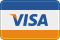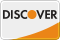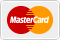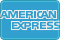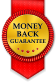# PHP 5.3.3 - Appending Well Formed XML Data WordPress

• SOLVED

Hello,

I am needing assistance with a PHP Script and I can not figure out how to finish it.

I have a script that gets fired off from a Flash Application about every 5 minutes upon completion of a specific event.

The PHP script generates 20 random numbers that gets saved to 2 different files. 1 file gets overwritten each time the script is run and the other get appended to the current data.

The format of the data file must be XML. I have a working script that generates 1 set of this data but I can not get the proper format on the appending of the data. It becomes malformed and the flash application errors out.

The script behavior is very simple.
If this data file does not exist, then the file gets created. The game number simply starts at 1 and then increments by 1 each time the script is run.

The number nodes are populated by a random sequence that can not have any duplicates. The range of numbers are from 1 to 80 where only 20 numbers get selected.

Below is an example of multiple calls to the script.

The XML data must be well formed using DOM classes. I believe my actionscript uses DOM standards as well so to stay solid, DOM in PHP should be used.

Thanks. I look forward to a resolution for this project!

The format that I need is as follows. This would be 3 instances that the script was run.
``` <?xml version="1.0"?> <Container> <Game> <gamenumber>1</gamenumber> <number>1</number> <number>2</number> <number>3</number> <number>4</number> <number>5</number> <number>6</number> <number>7</number> <number>8</number> <number>9</number> <number>10</number> <number>11</number> <number>12</number> <number>13</number> <number>14</number> <number>15</number> <number>16</number> <number>17</number> <number>18</number> <number>19</number> <number>20</number> </Game> <Game> <gamenumber>2</gamenumber> <number>1</number> <number>2</number> <number>3</number> <number>4</number> <number>5</number> <number>6</number> <number>7</number> <number>8</number> <number>9</number> <number>10</number> <number>11</number> <number>12</number> <number>13</number> <number>14</number> <number>15</number> <number>16</number> <number>17</number> <number>18</number> <number>19</number> <number>20</number> </Game> <Game> <gamenumber>3</gamenumber> <number>1</number> <number>2</number> <number>3</number> <number>4</number> <number>5</number> <number>6</number> <number>7</number> <number>8</number> <number>9</number> <number>10</number> <number>11</number> <number>12</number> <number>13</number> <number>14</number> <number>15</number> <number>16</number> <number>17</number> <number>18</number> <number>19</number> <number>20</number> </Game> </Container> ```

Here is my code that generated the first data set.
``` <?php //Create the random numbers \$balls = range(1,80); shuffle(\$balls); \$pick = array_slice(\$balls,1,20); //\$drawn = implode(", ",\$pick); \$arraySize = sizeof(\$pick); \$xml_file= 'allGames.xml'; \$drawn = array(); //Creates XML string and XML document using the DOM \$dom = new DomDocument('1.0'); //add root - <Container> \$container = \$dom->appendChild(\$dom->createElement('Container')); //add <Game> element to <Container> \$game = \$container->appendChild(\$dom->createElement('Game')); for (\$i=0; \$i!= \$arraySize; \$i++){ \$drawn [] = array( 'number' => \$pick[\$i], ); } //add <gamenumber> element to <Game> \$gamenumber = \$game->appendChild(\$dom->createElement('gamenumber')); //add <gamenumner> text node element to <gamenumber> \$gamenumber->appendChild( \$dom->createTextNode(\$gNumber+1)); foreach( \$drawn as \$draw ) //Populates the number element with the random array { //add <number> element to <Game> \$number = \$game->appendChild(\$dom->createElement('number')); //add <number> text node element to <number> \$number->appendChild( \$dom->createTextNode(\$draw['number'])); } //Generate the xml data files \$dom->formatOutput = true; // set the formatOutput attribute of // domDocument to true // save XML as two files //Appends to \$xml_file if it exists \$handle = fopen(\$xml_file, 'a+'); fwrite(\$handle, \$dom->saveXML()); fclose(\$handle); //Overwrites gameNumbers.xml \$handle = fopen('gameNumbers.xml', 'w'); fwrite(\$handle, \$dom->saveXML()); fclose(\$handle); echo \$dom->saveXML(); ?> ```

2010-08-13

You have to change the append part to something like this:

``` //Appends to \$xml_file if it exists \$handle = fopen(\$xml_file, 'a+'); fseek(\$handle,-12,SEEK_CUR); fwrite(\$handle, \$dom->saveXML()); fwrite(\$handle,"</Container>"); fclose(\$handle); ```

This does not work. I replaced the code that writes the files with yours. This is the output that I get. This would be considered malformed in the flash application.

We can't duplicate the xml version or the Container tags. The game number did not increment either.

Can you explain your thoughts on the fseek?

Ran the script once -
``` <?xml version="1.0"?> <Container> <Game> <gamenumber>1</gamenumber> <number>65</number> <number>58</number> <number>11</number> <number>17</number> <number>29</number> <number>74</number> <number>45</number> <number>57</number> <number>46</number> <number>16</number> <number>30</number> <number>66</number> <number>51</number> <number>59</number> <number>62</number> <number>2</number> <number>12</number> <number>7</number> <number>78</number> <number>34</number> </Game> </Container> <?xml version="1.0"?> <Container> <Game> <gamenumber>1</gamenumber> <number>65</number> <number>58</number> <number>11</number> <number>17</number> <number>29</number> <number>74</number> <number>45</number> <number>57</number> <number>46</number> <number>16</number> <number>30</number> <number>66</number> <number>51</number> <number>59</number> <number>62</number> <number>2</number> <number>12</number> <number>7</number> <number>78</number> <number>34</number> </Game> </Container> </Container> ```

Ran the script a second time
``` <?xml version="1.0"?> <Container> <Game> <gamenumber>1</gamenumber> <number>65</number> <number>58</number> <number>11</number> <number>17</number> <number>29</number> <number>74</number> <number>45</number> <number>57</number> <number>46</number> <number>16</number> <number>30</number> <number>66</number> <number>51</number> <number>59</number> <number>62</number> <number>2</number> <number>12</number> <number>7</number> <number>78</number> <number>34</number> </Game> </Container> <?xml version="1.0"?> <Container> <Game> <gamenumber>1</gamenumber> <number>65</number> <number>58</number> <number>11</number> <number>17</number> <number>29</number> <number>74</number> <number>45</number> <number>57</number> <number>46</number> <number>16</number> <number>30</number> <number>66</number> <number>51</number> <number>59</number> <number>62</number> <number>2</number> <number>12</number> <number>7</number> <number>78</number> <number>34</number> </Game> </Container> </Container><?xml version="1.0"?> <Container> <Game> <gamenumber>1</gamenumber> <number>47</number> <number>7</number> <number>15</number> <number>4</number> <number>60</number> <number>13</number> <number>66</number> <number>22</number> <number>6</number> <number>48</number> <number>5</number> <number>71</number> <number>65</number> <number>23</number> <number>40</number> <number>18</number> <number>31</number> <number>10</number> <number>54</number> <number>28</number> </Game> </Container> <?xml version="1.0"?> <Container> <Game> <gamenumber>1</gamenumber> <number>47</number> <number>7</number> <number>15</number> <number>4</number> <number>60</number> <number>13</number> <number>66</number> <number>22</number> <number>6</number> <number>48</number> <number>5</number> <number>71</number> <number>65</number> <number>23</number> <number>40</number> <number>18</number> <number>31</number> <number>10</number> <number>54</number> <number>28</number> </Game> </Container> </Container> ```

Also,
\
My understanding of the append attribute 'a+' that it always appends at the end of the file, no matter what?

Yes No?

Thanks.

With the fseek I try to rewrite the last </Container>

Try it:

``` //Appends to \$xml_file if it exists \$handle = fopen(\$xml_file, 'a+'); fseek(\$handle,-12,SEEK_END); \$xml = preg_replace('<?xml version="1.0"?>','',\$dom->saveXML()); \$xml = preg_replace('<Container>','',\$xml); fwrite(\$handle, \$xml); fwrite(\$handle,"</Container>"); fclose(\$handle); ```

And change the a+ with a:

``` //Appends to \$xml_file if it exists \$handle = fopen(\$xml_file, 'a'); fseek(\$handle,-12,SEEK_END); \$xml = preg_replace('<?xml version="1.0"?>','',\$dom->saveXML()); \$xml = preg_replace('<Container>','',\$xml); fwrite(\$handle, \$xml); fwrite(\$handle,"</Container>"); fclose(\$handle); ```

I replaced the writing the file code with the newest.

All I get now is
</Container>

When Run twice
</Container></Container>

The above duplication was my fault, I only commented one of the of the writing sections.

No matter how I play with this, I only get the closing container tag

Some improvements and fully tested!

``` //Appends to \$xml_file if it exists \$xml = \$dom->saveXML(); if (file_exists(\$xml_file)) { \$handle = fopen(\$xml_file, 'r+'); fseek(\$handle,-13,SEEK_END); \$xml = preg_replace('/<\?xml version="1.0"\?>/','',\$xml); \$xml = preg_replace('/<Container>/','',\$xml); } else { \$handle = fopen(\$xml_file, 'a+'); } fwrite(\$handle, \$xml); fclose(\$handle); ```

That's it!! I searched the net for almost a week for help on this and you nailed it about 15 minutes! My gamenumber is not incrementing but I can fix that.

One quick question, What is causing the the two blank lines in between the appended games sets?

``` <number>8</number> </Game> <Game> <gamenumber>2</gamenumber> <number>65</number> ```

Some new lines. Cleaned!

``` //Appends to \$xml_file if it exists \$xml = \$dom->saveXML(); if (file_exists(\$xml_file)) { \$handle = fopen(\$xml_file, 'r+'); fseek(\$handle,-14,SEEK_END); \$xml = preg_replace('/<\?xml version="1.0"\?>\n/','',\$xml); \$xml = preg_replace('/<Container>/','',\$xml); } else { \$handle = fopen(\$xml_file, 'a+'); } fwrite(\$handle, \$xml); fclose(\$handle); ```